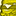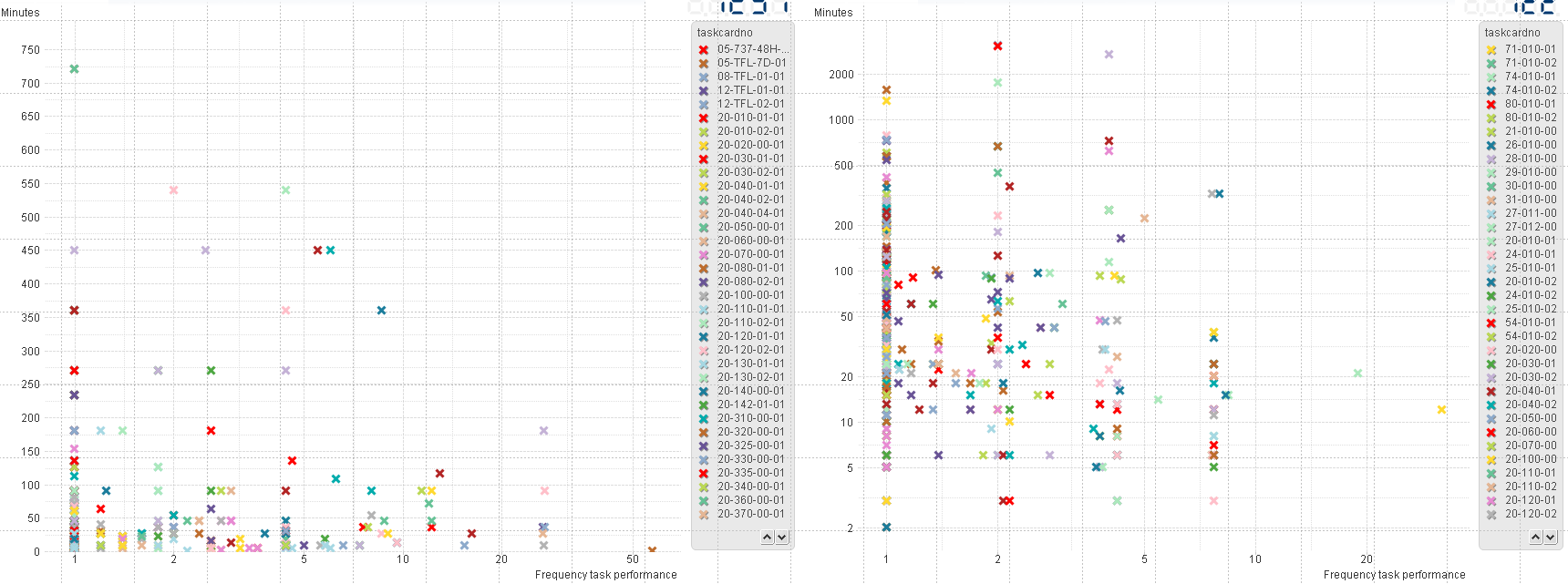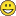# New to Qlik Sense

If you’re new to Qlik Sense, start with this Discussion Board and get up-to-speed quickly.

Announcements
Don't miss the upcoming Q&A with Qlik session on Qlik Application Automation on November 16th! REGISTER NOW
cancel
Showing results for
Did you mean:Not applicable

## Logaritmic scale, values disapear?

Hi guys, I've got a little issue with a scatter chart. I want to use a log scale for a scatter chart, it works for the x-axis. But when I gottle it for the y-axis all the values within my graph disappear. Any idea what could cause this behaviour?

For another graph, exactly the same, only data from another sample. It does work.

1 Solution

Accepted SolutionsCreator III

Can you change the y-axis expression to

Y-Axis: i=if(operator= 'AAA' AND total_hours>0 , total_hours , null())

And see if suppressing the zeroes works?  (If the expression above doesn't work you might just add a filter and see if manually suppressing the zeroes is the answer - or even taking them out of your data set to test).

9 RepliesSpecialist

Hello,Not applicable
AuthorLeft and right are exactly the same constructed. Same data source etc. The ONLY difference is that Operator is different for the other graph...

Expresions:

X-Axis: Count({<operator={'AAA'}>} DISTINCT status_remarks)/count({<operator={'AAA'}>}DISTINCT ac_registr)

Y-Axis: i=if(operator= 'AAA',total_hours)

dimension:

Calendaryear

total_hours are numbers. Status_remarks is a unique code which I use to count what task is performed on what aircraft and what date.

Now the left graph its Y-axis does not go logarithmic. When I check that box, the scatter points disappear. (no, they dont just go out of range)Creator III

What do you mean "all the values within my graph disappear"?  I can see values in both your screenshots.

Can you show just the left-hand chart data before & after log-axis is applied?  One thing might be zeroes in the data, but I don't think that will affect all the data points.Not applicable
Author

Yes because the Y-axis on the left graph is not set on a logarithmic scale.

If I do this, it will not go logaritmic. The axis stays the same. But the data points disapear.Creator III

Can you change the y-axis expression to

Y-Axis: i=if(operator= 'AAA' AND total_hours>0 , total_hours , null())

And see if suppressing the zeroes works?  (If the expression above doesn't work you might just add a filter and see if manually suppressing the zeroes is the answer - or even taking them out of your data set to test).Not applicable
Author

It works, thanks! What is the explanation behind this?

No null values are allowed or empty values?Creator III

I think zero values are not allowed (null values are allowed - they are ignored).

Log(0) is undefined, so the chart is just dropping all the data, not just the invalid data points. (Excel would just drop the invalid data points in this situation, you would still see the valid ones).

The formula just says "if total hours is zero, replace it will NULL()" and the NULL() is ignored on the chart.Not applicable
Author

How can i change below expression to suppress zero values:

sum({\$<DateType = {completion}, final_test_status={'passed'},

MonthPeriod1 = {"<\$(=Max(MonthPeriod1))"}>}course_completed)Creator III

I'm not very good at set expressions, so I will assume your expression is giving you the numerically correct results.

You have to wrap your expression in an IF() statement;

IF (Expression = 0 , null() , Expression)  (If Expression equals zero, then give a null, otherwise give the expression)

In your case it would be;

IF (sum({\$<DateType = {completion}, final_test_status={'passed'},

MonthPeriod1 = {"<\$(=Max(MonthPeriod1))"}>}course_completed) = 0 , null() ,

sum({\$<DateType = {completion}, final_test_status={'passed'},

MonthPeriod1 = {"<\$(=Max(MonthPeriod1))"}>}course_completed))Tags
Community Browser